jQuery插件开发方式主要有三种：

• 1、通过\$.extend()来扩展jQuery
• 2、通过\$.fn 向jQuery添加新的方法
• 3、通过\$.widget()应用jQuery UI的部件工厂方式创建

``````\$.extend({
sayHello: function(name) {
console.log('Hello,' + (name ? name: 'world') + '!');
}
})
\$.sayHello(); //调用
\$.sayHello('itmyhome'); //带参调用
``````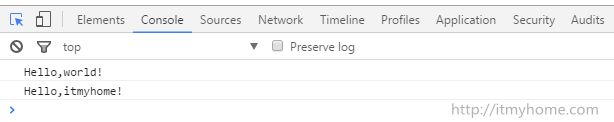``````\$.extend({
log: function(message) {
var now = new Date(),
y = now.getFullYear(),
m = now.getMonth() + 1, //！JavaScript中月分是从0开始的
d = now.getDate(),
h = now.getHours(),
min = now.getMinutes(),
s = now.getSeconds(),
time = y + '/' + m + '/' + d + ' ' + h + ':' + min + ':' + s;
console.log(time + ' My App: ' + message);
}
})
\$.log('initializing...'); //调用
``````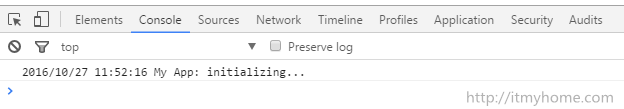#### 基本方法

``````\$.fn.pluginName = function() {
}
``````

``````<ul>
<li>
<p title="one">我是第一行</p>
</li>
<li>
<p title="two">我是第二行</p>
</li>
<li>
<p title="three">我是第三行</p>
</li>
<li>
<span> 我是span标签不是p标签哦 </span>
</li>
</ul>
``````
``````\$.fn.myPlugin = function() {
//在这里面,this指的是用jQuery选中的元素
//example :\$('p'),则this=\$('p')
this.css('color', 'red');
}

\$(function() {
\$('p').myPlugin();
})
``````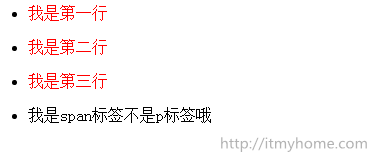``````\$.fn.myPlugin = function() {
//在这里面,this指的是用jQuery选中的元素
//example :\$('p'),则this=\$('p')
this.css('color', 'red');

this.each(function() {
//对每个元素进行操作
\$(this).append(' ' + \$(this).attr('title'));
})
}
``````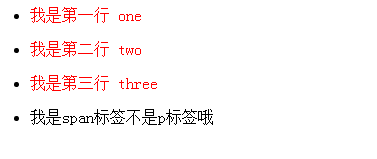#### 支持链式调用

``````\$.fn.myPlugin = function() {
//在这里面,this指的是用jQuery选中的元素
//example :\$('p'),则this=\$('p')
this.css('color', 'red');

return this.each(function() {
//对每个元素进行操作
\$(this).append(' ' + \$(this).attr('title'));
})
}
``````

#### 让插件接收参数

``````\$.fn.myPlugin = function(options) {
var defaults = {
'color' : 'red',
'fontSize' : '15px'
};

var settings = \$.extend(defaults, options);
return this.css( {
'color' : settings.color,
'fontSize' : settings.fontSize
});
}
``````

``````\$('p').myPlugin( {
'color' : '#668B8B'
});
``````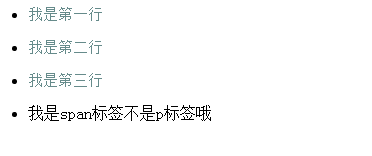``````\$('p').myPlugin( {
'color' : '#668B8B',
'fontSize': '20px'
});
``````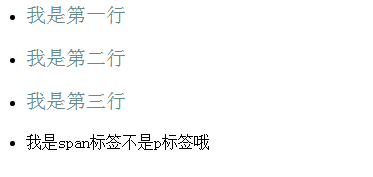#### 保护好默认参数

``````\$.fn.myPlugin = function(options) {
var defaults = {
'color' : 'red',
'fontSize' : '15px'
};

var settings = \$.extend({},defaults, options);
return this.css( {
'color' : settings.color,
'fontSize' : settings.fontSize
});

}
``````